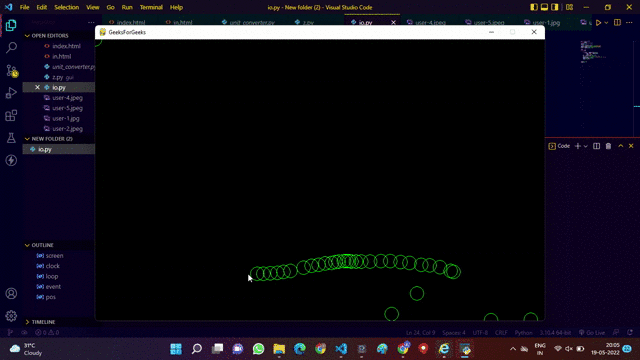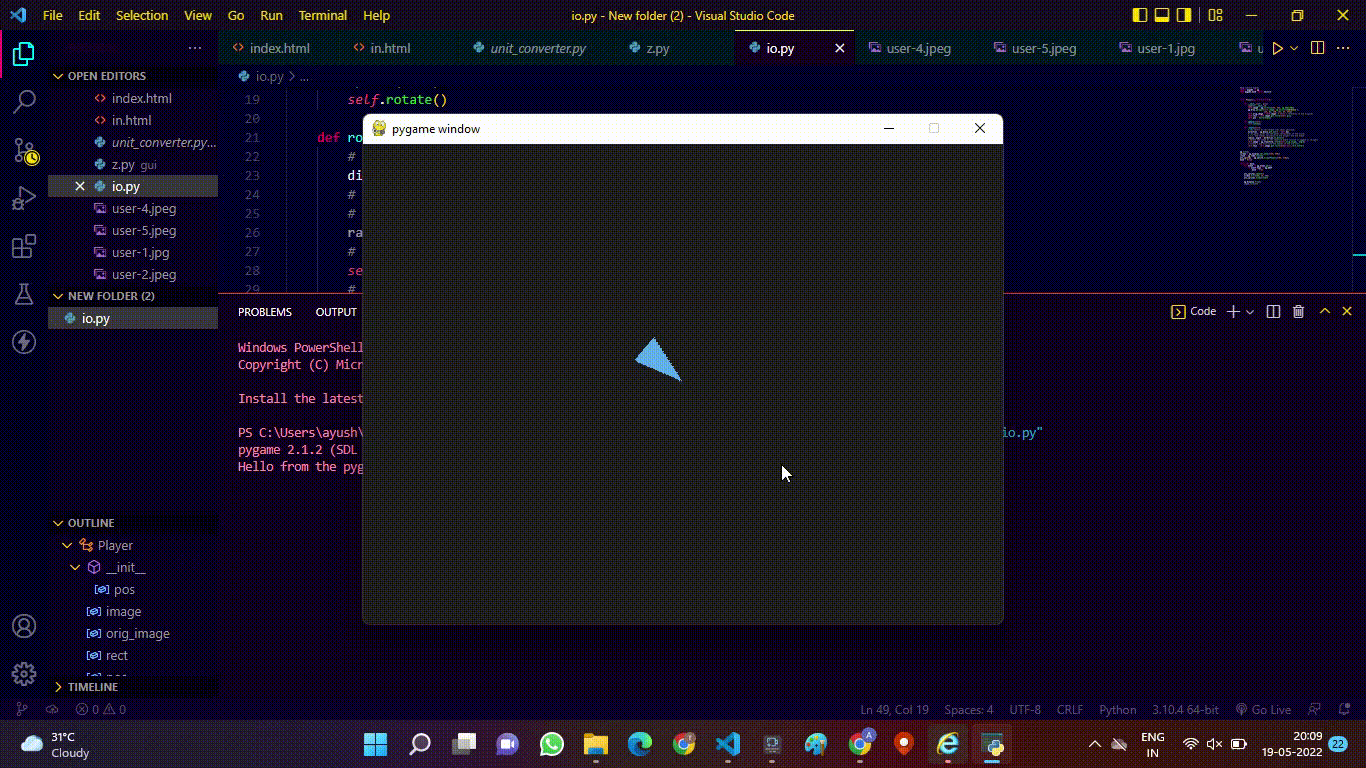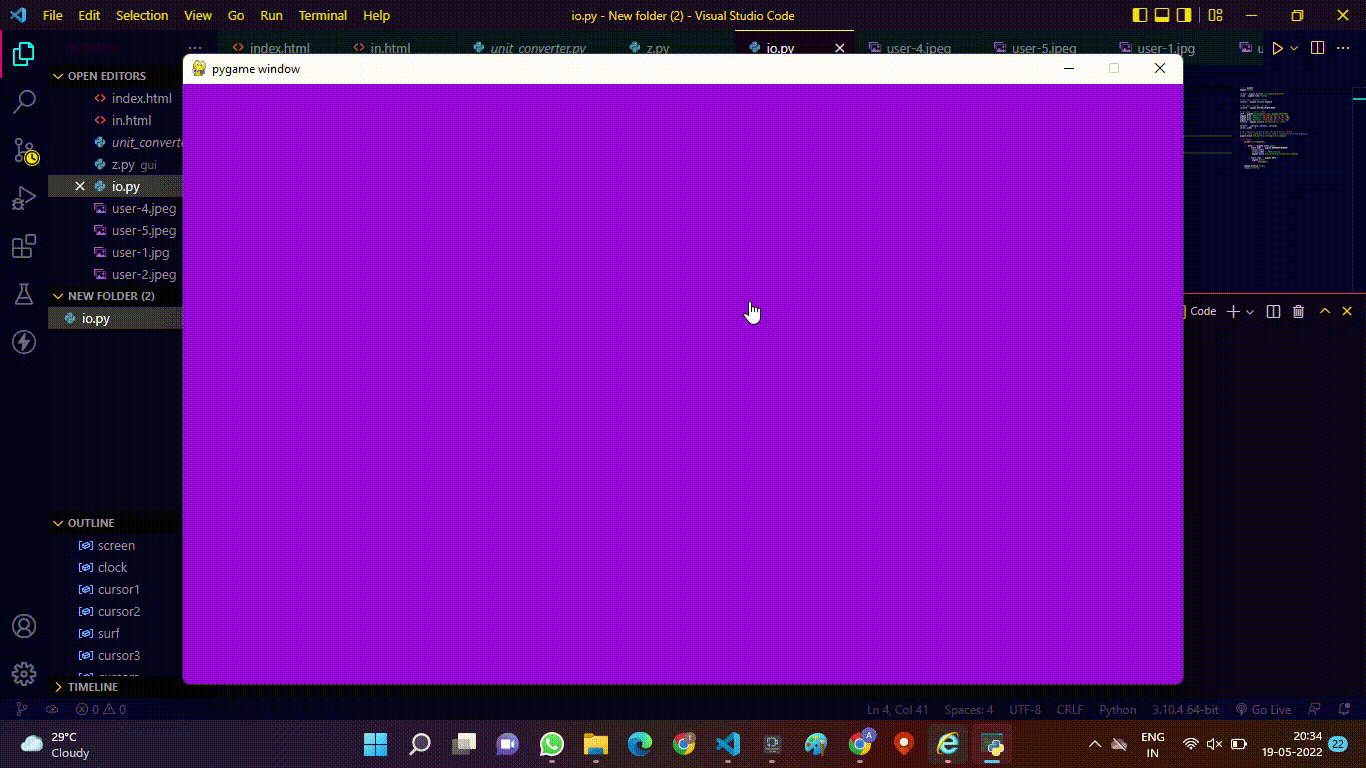# PyGame Set Mouse Cursor from Bitmap

• Last Updated : 22 Jun, 2022

In this article, we are going to see how to set the mouse cursor from bitmap using the PyGame module in Python.

### What is PyGame?

• It is a cross-platform set of Python modules designed for writing video games.
• It includes computer graphics and sound libraries designed to be used with the Python programming language.
• It can handle time, video, music, fonts, different image formats, cursors, mouse, keyboard, joysticks, etc.

### What is Bitmap?

• A bitmap is an array of binary data representing the values of pixels in an image.
• A GIF is an example of a graphics image file that has a bitmap.

## Mouse Function in PyGame

It is used in games where the program needs to stay updated regarding the position of the cursor.

#### pygame.mouse.get_pressed()

Returns the sequence of booleans representing the state of all the mouse buttons. A true value means the mouse is currently being pressed at the time of the call.

Syntax: pygame.mouse.get_pressed(num_buttons=3) -> (button1, button2, button3)

Parameter:

• num_buttons : Number of buttons in mouse (default value = 3).

#### pygame.display.set_mode()

This function is used to create a display surface. The arguments passed in are requests for a display type. The actual created display will be the best possible match supported by the system.

Syntax : pygame.display.set_mode(resolution=(0,0), flags=0, depth=0)

Parameter:

• Resolution : A pair of numbers representing the width and height of  the window.
• Flags : Additional options that change the type of window.
• Depth : Amount of bits used for color.

#### pygame.mouse.get_pos()

This is used to get the X and Y coordinates of the mouse cursor. These coordinates are relative to the top-left corner of the display. The cursor position is relative

Syntax : pygame.mouse.get_pos()

Return: Coordinates of mouse are stored.

#### pygame.quit()

This is used to shutdown the game.

Syntax : pygame.quit()

#### clock.tick()

By calling clock.tick(60) once per frame, the program will never run at more than 60 frames per second.

Syntax : clock.tick(framerate=0)

Parameter :

• Framerate : It will compute how many milliseconds have passed since the previous call.

## Example 1: Creating the main.py file to create a circle on the mouse path.

In this example, We are going to draw a circle on the screen wherever the cursor moves inside the window, and to implement this we have to follow the following steps:

• We will be making a canvas of size 960*600.
• Writing code to make our circle.
• Keep a track of the mouse cursor within the canvas.
• Drawing circles in the places wherever the cursor is moving.

## Python3

 `import` `pygame``# initializing the pygame``pygame.init()``# displaying Canvas (960*600)``screen ``=` `pygame.display.set_mode((``960``, ``600``))``pygame.display.set_caption(``'GeeksForGeeks'``)``clock ``=` `pygame.time.Clock()`` ` `loop ``=` `True``while` `loop:`` ` `    ``for` `event ``in` `pygame.event.get():``        ``if` `event.``type` `=``=` `pygame.QUIT:``            ``loop ``=` `False``    ``pos ``=` `pygame.mouse.get_pos()``    ``# giving color and shape to the circle``    ``pygame.draw.circle(screen, (``0``, ``255``, ``0``),``                       ``pos, ``15``, ``1``)``    ``pygame.display.update()``    ``clock.tick(``60``)`` ` ` ` `pygame.quit()``# quit()`

Output:## Example 2: Creating the main.py file to locate the direction of the cursor.

In this example, We are going to draw an arrow in the center of the window and this arrow is pointing towards the cursor wherever the cursor moves inside the canvas window to implement this we have to follow the following steps:

• We will be making a canvas of size 640*480.
• Now write a code to draw an arrow that points toward the pointer of the mouse.
• Now make a program to rotate the arrow towards the cursor.

## Python3

 `import` `pygame as pg``from` `pygame.math ``import` `Vector2`` ` ` ` `class` `Player(pg.sprite.Sprite):``   ``# main function starts here``    ``def` `__init__(``self``, pos):``        ``super``().__init__()``        ``self``.image ``=` `pg.Surface((``50``, ``30``),``                                ``pg.SRCALPHA)``        ``pg.draw.polygon(``self``.image,``                        ``pg.Color(``'steelblue2'``),``                        ``[(``0``, ``0``), (``50``, ``15``), (``0``, ``30``)])``        ``self``.orig_image ``=` `self``.image``        ``# Store a reference to the original.``        ``self``.rect ``=` `self``.image.get_rect(center``=``pos)``        ``self``.pos ``=` `Vector2(pos)`` ` `    ``def` `update(``self``):``        ``self``.rotate()``# Rotate the arrow function`` ` `    ``def` `rotate(``self``):``        ``# The vector to the target (the mouse position).``        ``direction ``=` `pg.mouse.get_pos() ``-` `self``.pos``        ``# .as_polar gives you the polar``        ``# coordinates of the vector,``        ``# i.e. the radius (distance to the target)``        ``# and the angle.``        ``radius, angle ``=` `direction.as_polar()``        ``# Rotate the image by the negative angle``        ``# (y-axis in pygame is flipped).``        ``self``.image ``=` `pg.transform.rotate(``self``.orig_image,``                                         ``-``angle)``        ``# Create a new rect with the``        ``# center of the old rect.``        ``self``.rect ``=` `self``.image.get_rect(center``=``self``.rect.center)`` ` ` ` `pg.init()``# Creating a canvas of size 640*480``screen ``=` `pg.display.set_mode((``640``, ``480``))``clock ``=` `pg.time.Clock()``all_sprites ``=` `pg.sprite.Group(Player((``300``, ``220``)))``done ``=` `False`` ` `while` `not` `done:``    ``for` `event ``in` `pg.event.get():``        ``if` `event.``type` `=``=` `pg.QUIT:``            ``done ``=` `True`` ` `    ``all_sprites.update()``    ``screen.fill((``30``, ``30``, ``30``))``    ``all_sprites.draw(screen)`` ` `    ``pg.display.flip()``    ``clock.tick(``30``)`

Output:## Example 3: Changing the cursor shape of the mouse

In this example, We are going to change the shape of the cursor whenever we clicked on the mouse if the cursor is inside the screen window to implement this we have to follow the following steps:

• First, we will make a canvas 600*400.
• White a code to track the cursor of the mouse.
• Now we will write a code to change the shape of the Cursor if mouse is clicked.

## Python3

 `import` `pygame``pygame.init()`` ` `# Creating a canvas of 600*400``screen ``=` `pygame.display.set_mode((``600``, ``400``))``clock ``=` `pygame.time.Clock()`` ` `# old type, "bitmap" cursor``cursor1 ``=` `pygame.cursors.diamond`` ` `# new type, "system" cursor``cursor2 ``=` `pygame.SYSTEM_CURSOR_HAND`` ` `# new type, "color" cursor``surf ``=` `pygame.Surface((``30``, ``25``), pygame.SRCALPHA)``pygame.draw.rect(surf, (``0``, ``255``, ``0``), [``0``, ``0``, ``10``, ``10``])``pygame.draw.rect(surf, (``0``, ``255``, ``0``), [``20``, ``0``, ``10``, ``10``])``pygame.draw.rect(surf, (``255``, ``0``, ``0``), [``5``, ``5``, ``20``, ``20``])``cursor3 ``=` `pygame.cursors.Cursor((``15``, ``5``), surf)`` ` `cursors ``=` `[cursor1, cursor2, cursor3]``cursor_index ``=` `0`` ` `# the arguments to set_cursor can be a Cursor object``# or it will construct a Cursor object``# internally from the arguments``pygame.mouse.set_cursor(cursors[cursor_index])`` ` `while` `True``:``    ``screen.fill(``"purple"``)`` ` `    ``for` `event ``in` `pygame.event.get():``        ``if` `event.``type` `=``=` `pygame.MOUSEBUTTONDOWN:``            ``cursor_index ``+``=` `1``            ``cursor_index ``%``=` `len``(cursors)``            ``pygame.mouse.set_cursor(cursors[cursor_index])`` ` `        ``if` `event.``type` `=``=` `pygame.QUIT:``            ``pygame.quit()``            ``raise` `SystemExit`` ` `    ``pygame.display.flip()``    ``clock.tick(``144``)`

Output:My Personal Notes arrow_drop_up